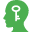# Embedded Figures

In a Competitive exam, Reasoning is one of the most important section. A good score in Reasoning test can lead you to score very good marks in competitive exams. You can achieve a good marks only if you have a depth knowledge of reasoning skills. Basically reasoning is divided into few sections according to logic and type. This Embedded Figures topic is one of them.

"A figures is said to be embedded in another figure when the second figure completely contains the first figure."

Different types of questions covered in this section are as follows

1. Question Figure Embedded in Answer Figure
2. Answer Figure Embedded in Question Figure

Questions based on Embedded Figures comprise of a question figure and four answer figures and it is asked to find the correct answer figure embedded in given question figure or the correct answer figure in which the given question figure is embedded.

These type of problems judge the visual ability and concentration level of a candidate. While attempting these questions, the candidates should visually judge the figure and try to find out the option in which this figure is embedded.

Let us consider following two figures

You are required to find out if Fix. (X) is embedded on Fig (Y).By careful examination of Fig (Y), we find that Fig (X) is embedded in it, as shown in the below figure.## Types of problems which are asked in various competitive examinations are given below

Tip#1 : Question Figure Embedded in Answer Figure

In such questions, a question figure and four answer figures are given. The question figure is embedded in one of the answer figures. The candidate is required to find out that particular answer figure in which the question figure is embedded.Memory Add-ons

1. There may be some questions in which the question figure is not directly embedded in any of the answer figures. In these type of questions, change the orientation of question figure to find the correct answer figure.
2. In some questions, the candidate will find the question figure embedded in two or more answer figures; then the most appropriate answer is that in which the question figure is embedded with least change in its orientation.

Directions (Example Nos. 1-5)In the following questions, a question figure and a set of four answer figures (a), (b), (c) and (d) are given. Find out that answer figure in which the question figure is embedded.

Example 1: Find out that answer figure in which the question figure is embedded.Solution: (a) Clearly, the question figure is embedded in answer figure (a). The portion which question figure occupies in the alternative figure has been shown in the below figure.Example 2: Find out that answer figure in which the question figure is embedded.Solution: (d) Clearly, the question figure is embedded in answer figure (d) only.Example 3: Find out that answer figure in which the question figure is embedded.Solution: (d) Clearly, the question figure is embedded in answer figure (d) only.